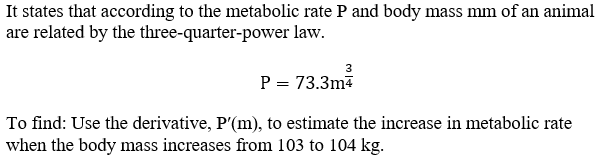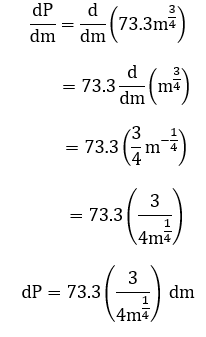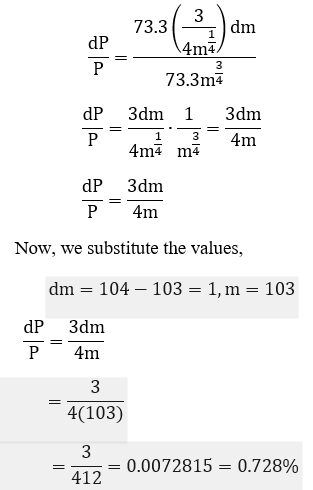# According to Kleiber’s Law, the metabolic rate P (in kilocalories per day) and body mass mm (in kilograms) of an animal are related by the three‑quarter‑power law P=73.3m^3/4. Use the derivative, P′(m), to estimate the increase in metabolic rate when the body mass increases from 103 to 104 kg.(Use decimal notation. Give your answer to three decimal places.)Metabolic rate increases by approximately kcal/day?

Question
85 views

According to Kleiber’s Law, the metabolic rate P (in kilocalories per day) and body mass mm (in kilograms) of an animal are related by the three‑quarter‑power law P=73.3m^3/4. Use the derivative, P′(m), to estimate the increase in metabolic rate when the body mass increases from 103 to 104 kg.

Metabolic rate increases by approximately kcal/day?
check_circle

Given Kleiber’s Law as below:We find P′(m)We find the percentage change as follows:...

### Want to see the full answer?

See Solution

#### Want to see this answer and more?

Solutions are written by subject experts who are available 24/7. Questions are typically answered within 1 hour.*

See Solution
*Response times may vary by subject and question.
Tagged in